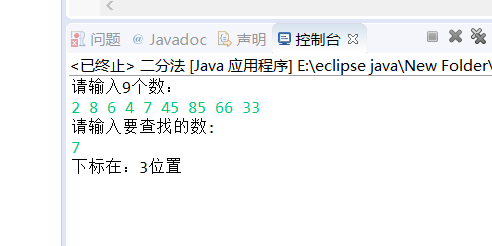# 小强哥in资料

1.

（1）使用这种方法首先要对元素进行排序；

（2）之后要确定元素的中间位置m；

（3）然后让要查询的数于中间的数进行比较，如果中间的数比要查询的数大，那么m-1变成最大值，否则让m+1变成最小值；

`package main函数; import java.util.Scanner; public class 二分法 { 	public static void main(String[]args) 	{ 		Scanner input = new Scanner(System.in); 		System.out.println("请输入9个数："); 		int[] m=new int; 		for(int i=0;i<9;i++) 		{ 			m[i] = input.nextInt(); 		} 		int temp; 		for(int i=0;i<m.length-1;i++) 			for(int j=i+1;j<m.length;j++) 			{ 				if(m[i]>m[j]) 				{ 					temp=m[i]; 					m[i]=m[j]; 					m[j]=temp; 				} 			} 		System.out.println("请输入要查找的数:"); 		int value=input.nextInt(); 		int cout=-1; 		int low=0; 		int high=m.length-1; 		while(low<=high) 		{ 			int mid=(low+high)/2; 			if(m[mid]==value) 			{ 				cout=mid; 				break; 			} 			else if(m[mid]>value) 			{ 				high=mid-1; 			} 			else 			{ 				low=mid+1; 			} 		} 		if(cout!=-1) 			System.out.println("下标在："+cout+"位置"); 		else 			System.out.println("没有找到"); 		 	}  } `2.

java的一些工具类

（1）打印数组    toStrng方法打印数组

import java.util.Arrays;

int [] ary={元素………}

System out.println(Arrays.toString(ary));

（2）sort方法排序   //升序

import java.util.Arrays;

import java.util.Arrays;

int [] ary={元素………}

Arrays.sort(ary);

(3)二分查找

import java.util.Arrays;

binarySearch(int[] args,int key)

int [] ary={元素………}

Arrays.sort(ary);

int index=Arrays.binarySearch(ary,查找的数)；

（4）随机数

import java.util.Random;

Random ran=new Random;

int a=ran.nextInt(100);

(5)输入

import java.util.Scanner;
Scanner input = new Scanner(System.in);

qklbishe.com区块链毕设代做网专注|以太坊fabric-计算机|java|毕业设计|代做平台 » 小强哥in资料